# Divergence

• I
For the vector valud function F in the image, the three components of the output vector at a point are functions of (x,y,z)the three coordinates of the point.But while calculating divergence, why is the rate of change of x component of the output along x direction alone is accounted(similarly for y and z components)?Why isnt the change of x component along y and z directions taken into account?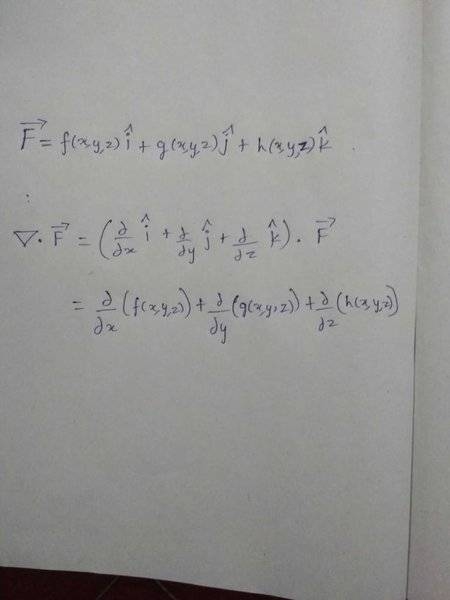#### Attachments

Mark44
Mentor
f(x, y, z) is already the component of the vector field F in the x direction, g(x, y, z) is the component in the y direction, and h(x, y, z) is the component in the z direction. By definition, the divergence of F, ##\nabla F## is the dot product of the three partials with the components of F.

Why isnt the change of x component along y and z directions taken into account?
The best answer I can come up with is that that's how divergence is defined.

•Mohankpvk and vela
f(x, y, z) is already the component of the vector field F in the x direction, g(x, y, z) is the component in the y direction, and h(x, y, z) is the component in the z direction. By definition, the divergence of F, ##\nabla F## is the dot product of the three partials with the components of F.

The best answer I can come up with is that that's how divergence is defined.
Ok.But from what ever I can understand, divergence calculates the total rate of change of the vector function as we move through the point from all the direction(i.e sum of change at the point along each direction).If this total is positive then the point is source,negative sink and zero -equal flux moves in and out.
How does divergence calculates this without accounting the change of the components in the other two directions(other than the components direction) as we pass through the point?

Mark44
Mentor
How does divergence calculates this without accounting the change of the components in the other two directions
Because each of the component functions (f, g, and h in your example) is in a single direction. I think you might be confusing the x, y, and z parameters of the component functions with the unit direction vectors ##\hat i, \hat j,## and ##\hat k##. Since, for example, f(x, y, z) is the component in the x direction at a point P(x, y, z), it doesn't make sense to me to calculate ##\frac{\partial}{\partial y}f(x, y, z)## or the partial of this function with respect to z. Similar for the other two component functions g and h.

•Mohankpvk
Stephen Tashi
Why isnt the change of x component along y and z directions taken into account?

Perhaps we can figure that out by taking it into account.

http://mathworld.wolfram.com/Divergence.html takes the approach used in physics:

The divergence of a vector field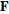, denoted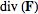or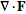(the notation used in this work), is defined by a limit of the surface integral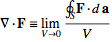where the surface integral gives the value ofintegrated over a closed infinitesimal boundary surface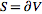surrounding a volume element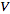, which is taken to size zero using a limiting process.

From the viewpoint of doing rigorous mathematics, making that definition precise would require some work!

Wolfram's abbreviated derivation of the divergence in Cartesian coordinates is:

A formula for the divergence of a vector field can immediately be written down in Cartesian coordinates by constructing a hypothetical infinitesimal cubical box oriented along the coordinate axes around an infinitesimal region of space. There are six sides to this box, and the net "content" leaving the box is therefore simply the sum of differences in the values of the vector field along the three sets of parallel sides of the box. Writing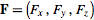, it therefore following immediately that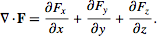To explain that proof at the level of a physics textbook, we can consider the cube to be small enough that the components of the vector field within are sufficiently approximated by linear functions.

For example, for the x-component of ##\overrightarrow{F}##, we assume:
## F_x( x_0 + x, y_0 + y, z_0 + z ) = F_x(x_0,y_0,z_0) + \frac{\partial F_x}{\partial x}| _{p_0} x + \frac{\partial F_x}{\partial y}|_{p_0}y + \frac{\partial F_x}{\partial z}|_{p_0} z ##.
where ##p_0 = (x_0,y_0,z_0)## is the point where we wish to compute the divergence of ##F##

For simplicity of notation, I will abbreviate that as
##F_x = F_{x_0} + Ax + By + Cz##

We compute the flux through a cube with sides ##\triangle s## centered on ##(x_0,y_0,z_0)## with edges parallel to the coordinate axes.

Consider computing the flux through the two faces of the cube that lie in the y-z plane. This involves using the unit normal vectors to those faces, which are (-1,0,0) and (1,0.0). For the face with normal (-1,0,0) the integration is
##\int_{y_0 -\triangle s/2}^{y_0 + \triangle s/2} \int_{z_0 - \triangle s/2}^{z_0 + \triangle s/2} (-1) ( F_{x_0} +A(- \triangle s/2) + B y + Cz)\ dz dy ##

## = \int_{y0 - \triangle s/2}^{y_0 + \triangle s/2} ( -F_{x_0} \triangle s + A (\triangle s/2) (\triangle s) - B y \triangle s - C (\big{|}_{z_0 - \triangle s/2}^ {z_0 + \triangle s/2} z^2/2) \ dy ##

## = \int_{y0 - \triangle s/2}^{y_0 + \triangle s/2} ( -F_{x_0} \triangle s + A (\triangle s/2) (\triangle s) - B y \triangle s - C 2 z_0 \triangle s/2) dy ##

## = (- F_{x_0}\triangle s) \triangle s + (A(\triangle s/2)(\triangle s))\triangle s - (B \triangle s) (2y_0 \triangle s)/2 - (C 2 z_0 \triangle s/2)(\triangle s) ##

## = -F_{x0} \triangle s^2 + A \triangle s^3/2 - By_0 \triangle s^2 - C z_0 \triangle s^2 ##

For the face with normal (1,0,0), the integration is
##\int_{y_0 -\triangle s/2}^{y_0 + \triangle s/2} \int_{z_0 - \triangle s/2}^{z_0 + \triangle s/2} (1) ( F_{x0} + A \triangle s/2 + B y + Cz)\ dz dy ##

## = F_{x0} \triangle s^2 + A \triangle s^3/2 + By_0 \triangle s^2+ C z_0 \triangle s^2 ##

Adding the results for the two faces, the total flux in the x-direction is

## 2 A \triangle s^3/2 = A \triangle s^3 = \frac{\partial F_x}{\partial x} | _{p_0} \triangle s^3##.

By similar calculations for the flux in the y and z direction the total flux through the cube is:

##\frac{\partial F_x}{\partial x} | _{p_0} \triangle s^3 + \frac{\partial F_y}{\partial y}|_{p_0} \triangle s^3 + \frac{\partial F_z}{\partial z}|_{p_0} \triangle s^3 ##

To obtain the divergence, we divide the total flux by the volume ##\triangle s^3## of the cube, so
## Div\ F(x,y,z)| _{(x_0,y_0,z_0)} =
\frac{\partial F_x}{\partial x} | _{p_0} + \frac{\partial F_y}{\partial y}|_{p_0} + \frac{\partial F_z}{\partial z}|_{p_0} ##

#### Attachments

Last edited:
•Mohankpvk
Stephen Tashi
Ok.But from what ever I can understand, divergence calculates the total rate of change of the vector function as we move through the point from all the direction(i.e sum of change at the point along each direction).

You need a better understanding. ( For that, you need to understand flux and surface integrals.)

Averages defined for things of finite extent and can be extended to concepts that apply to points - i.e. to things have zero extent. For example, the average mass per unit volume of an object of finite extent is defined. We extend this concept to the mass density of the object at a point (x,y,z) by considering a small cube with (x,y,z) at its center. Its average mass density is = mass in cube/ volume of cube. Then we take the limit of this ratio as the volume of the cube approaches zero.

Imagine F(x,y,z) is a vector field describing the flow of a gas. Something of finite extent (like a room) may be generating gas. For the room, we can define the average amount of gas generated per unit volume as = net amount of gas leaving the room divided by volume of the room. We can extend this concept to the intensity of gas generationat a point (x,y,z) in the room by considering a small cube with (x,y,z) at its center. The average gas per volume generated by the cube = net amount of gas leaving the cube divided by volume of the cube. We take the limit of this ratio as the volume of the cube approaches zero. That's the basic idea of the divergence of F.

•Mohankpvk
Perhaps we can figure that out by taking it into account.

http://mathworld.wolfram.com/Divergence.html takes the approach used in physics:

From the viewpoint of doing rigorous mathematics, making that definition precise would require some work!

Wolfram's abbreviated derivation of the divergence in Cartesian coordinates is:

To explain that proof at the level of a physics textbook, we can consider the cube to be small enough that the components of the vector field within are sufficiently approximated by linear functions.

For example, for the x-component of ##\overrightarrow{F}##, we assume:
## F_x( x_0 + x, y_0 + y, z_0 + z ) = F_x(x_0,y_0,z_0) + \frac{\partial F_x}{\partial x}| _{p_0} x + \frac{\partial F_x}{\partial y}|_{p_0}y + \frac{\partial F_x}{\partial z}|_{p_0} z ##.
where ##p_0 = (x_0,y_0,z_0)## is the point where we wish to compute the divergence of ##F##

For simplicity of notation, I will abbreviate that as
##F_x = F_{x_0} + Ax + By + Cz##

We compute the flux through a cube with sides ##\triangle s## centered on ##(x_0,y_0,z_0)## with edges parallel to the coordinate axes.

Consider computing the flux through the two faces of the cube that lie in the y-z plane. This involves using the unit normal vectors to those faces, which are (-1,0,0) and (1,0.0). For the face with normal (-1,0,0) the integration is
##\int_{y_0 -\triangle s/2}^{y_0 + \triangle s/2} \int_{z_0 - \triangle s/2}^{z_0 + \triangle s/2} (-1) ( F_{x_0} +A(- \triangle s/2) + B y + Cz)\ dz dy ##

## = \int_{y0 - \triangle s/2}^{y_0 + \triangle s/2} ( -F_{x_0} \triangle s + A (\triangle s/2) (\triangle s) - B y \triangle s - C (\big{|}_{z_0 - \triangle s/2}^ {z_0 + \triangle s/2} z^2/2) \ dy ##

## = \int_{y0 - \triangle s/2}^{y_0 + \triangle s/2} ( -F_{x_0} \triangle s + A (\triangle s/2) (\triangle s) - B y \triangle s - C 2 z_0 \triangle s/2) dy ##

## = (- F_{x_0}\triangle s) \triangle s + (A(\triangle s/2)(\triangle s))\triangle s - (B \triangle s) (2y_0 \triangle s)/2 - (C 2 z_0 \triangle s/2)(\triangle s) ##

## = -F_{x0} \triangle s^2 + A \triangle s^3/2 - By_0 \triangle s^2 - C z_0 \triangle s^2 ##

For the face with normal (1,0,0), the integration is
##\int_{y_0 -\triangle s/2}^{y_0 + \triangle s/2} \int_{z_0 - \triangle s/2}^{z_0 + \triangle s/2} (1) ( F_{x0} + A \triangle s/2 + B y + Cz)\ dz dy ##

## = F_{x0} \triangle s^2 + A \triangle s^3/2 + By_0 \triangle s^2+ C z_0 \triangle s^2 ##

Adding the results for the two faces, the total flux in the x-direction is

## 2 A \triangle s^3/2 = A \triangle s^3 = \frac{\partial F_x}{\partial x} | _{p_0} \triangle s^3##.

By similar calculations for the flux in the y and z direction the total flux through the cube is:

##\frac{\partial F_x}{\partial x} | _{p_0} \triangle s^3 + \frac{\partial F_y}{\partial y}|_{p_0} \triangle s^3 + \frac{\partial F_z}{\partial z}|_{p_0} \triangle s^3 ##

To obtain the divergence, we divide the total flux by the volume ##\triangle s^3## of the cube, so
## Div\ F(x,y,z)| _{(x_0,y_0,z_0)} =
\frac{\partial F_x}{\partial x} | _{p_0} + \frac{\partial F_y}{\partial y}|_{p_0} + \frac{\partial F_z}{\partial z}|_{p_0} ##
Thank you.Nice answer.So the two faces of the cube contain the points with the same y,z coordinates but different x coordinates.So as we move along x there will not be any change in the x component of the field over the face w.r.t y and z(since only x value changes).The only change is with respect to x.So the gradient accounts only the change w.r.t x.Is this interpretation right?
Is there any book(not text books) for vector calculus where I could get the intuitions or physical meanings of curl,gradient,ect..?

Stephen Tashi
TSo the gradient accounts only the change w.r.t x.Is this interpretation right?
Do you mean "gradient" or do you mean "divergence"?

Yes, the partial derivatives used in defining the gradient are involved in computing the divergence, but "gradient" and "divergence" are different things.

Yes, for a vector field whose component functions are linear in (x,y,z) only the term involving ##\frac{\partial F_x}{\partial x}## has a net contribution to computing the flux through the cube in the x-direction.

Is there any book(not text books) for vector calculus where I could get the intuitions or physical meanings of curl,gradient,ect..?

A book explaining the concepts of vector calculus well must resemble a text book.

I'm not familiar with modern texts. A long time ago, I read "Electromagnetic Fields and Waves" by Vladimir Rojansky, published by Dover Books. It has an intuitive introduction to the concepts of vector calculus. The book "DIV, Grad, Curl, and all that" by H.M. Schey has a reputation for being a good intuitive introduction to vector calculus, but I haven't read it. There are several editions of that book.

•Mohankpvk
Do you mean "gradient" or do you mean "divergence"?
Sorry.I meant divergence.

Thank you.